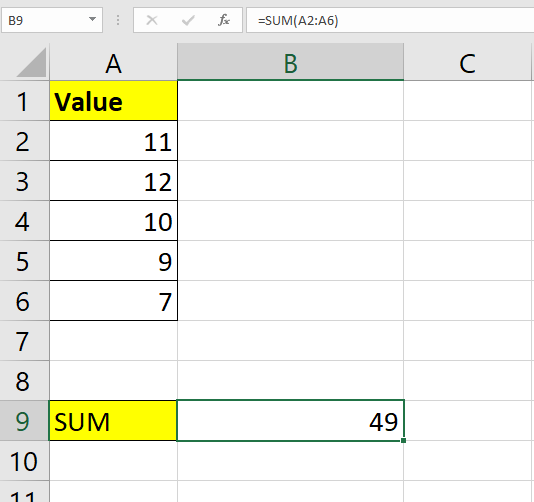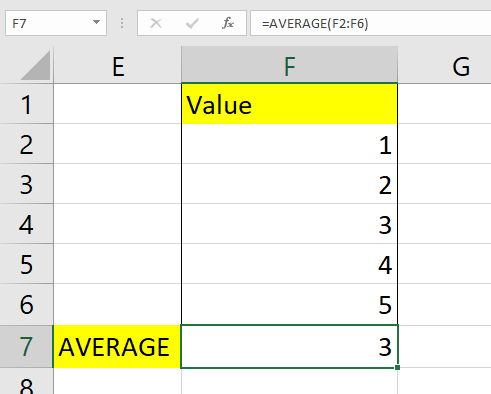# How to Fix Formula Not Updating Automatically in Excel

This tutorial will explain a few ways to fix formulas not updating automatically in Microsoft Excel.

By default, user-inputted formulas should update and evaluate on their own. If the formula does not evaluate, there may be an issue with the formula itself or a particular setting in your Excel program.

One possible reason why your Excel formulas are not updating is that your calculation settings have been configured to ‘Manual’. This application-level setting modifies how your cells are calculated.

Excel comes with three types of calculations. The automatic calculation indicates that Excel will recalculate all dependent formulas when we change a particular cell. Excel also gives us the option ‘Automatic Except for Data Tables’. These data tables can be found under the What-If Analysis option under the Data tab.

The last calculation option in Excel is called ‘Manual’. With this option selected, Excel will only recalculate cells when told to explicitly. The key F9 is the keyboard shortcut for recalculating the entire workbook. We can also use Shift + F9 to recalculate only the current worksheet.

Besides Excel calculation settings, the formula itself may have some issues preventing it from updating automatically. We will tackle a few of these other scenarios in the next section.

## A Real Example of Formulas Not Updating Automatically in Excel

Let’s take a look at a few real examples of cells in Excel that have an issue updating.

In the example below, we have a table of values we would like to sum together with the `SUM` function. However, despite placing the proper syntax, the formula still refuses to evaluate.Upon closer inspection, you notice that there is a blank space right before the ‘=’ character. Because of the extra character, the formula is treated as a string instead.

To evaluate the formula above, we simply need to remove the unnecessary blank space before ‘=’.

In the second example below, we have a series of values that we want to find the average of. We can use the `AVERAGE` function to compute this value.However, the formula returns a 0 instead of the actual average. In this case, the formula is not returning the actual result because of a circular reference.

The formula used in cell F7 is `=AVERAGE(F2:F7)`. Circular references evaluate to 0 by default in Microsoft Excel. To fix this issue, we can change the range to F2:F6 to avoid the circular reference.

Now that we know some common reasons why Excel formulas stop updating automatically, let’s learn how to address these issues. If you need to fix your own spreadsheet formulas in Excel, follow our guide in the next section!

## How to Fix Formula Not Updating Automatically in Excel

This section will guide you through each step needed to fix formulas that are not updating automatically. You’ll learn how to switch from Manual to Automatic calculation mode. You’ll also learn how to handle circular references and cases where Excel reads your formula as a normal string.

1. First, check if your formula contains unnecessary spaces. In the example below, the whitespace before our ‘=’ symbol prevents the formula from evaluating.2. After removing the leading space, hit the Enter key to evaluate the function.3. If your function returns a 0, you may have to fix a circular reference. By default, Excel will warn you whether a formula contains a circular reference. However, your application may have a different configuration.4. Remove the circular reference by excluding the current cell from any input. In the example below, we changed our cell range input from F2:F7 to F2:F6.If the above methods did not succeed in updating your formula, you may also check if your Excel program is set to an Automatic Calculation.

1. Navigate to the File tab found on the upper-left side of the screen.2. In the File tab, click on the Options button on the page’s lower-left side.3. A dialog box will appear labeled Excel Options. Click on the Formulas tab and look for the section title Calculation options. Ensure that the Workbook Calculation option is set to Automatic. Click on the OK button to apply these changes.1. What is the purpose of using Manual mode?
One major reason why users select Manual calculation is to help handle worksheets with large amounts of data. With large files, it may take some time to update every cell when we make changes to the data. Switching to Manual mode can help avoid slowing down your system.

This step-by-step guide should help you fix formulas that are not updating automatically in Microsoft Excel.

We’ve shown how to adjust the calculation mode of your Excel application. You should now know how to handle formulas set as text and formulas with circular references.

With this guide, you should now be able to troubleshoot most formulas in Microsoft Excel that refuse to update automatically. With so many other Microsoft Excel functions available, you can surely find one that suits your use case.

Are you interested in learning more about what Microsoft Excel can do? Subscribe to our newsletter to find out about the latest Microsoft Excel guides and tutorials from us.### Get emails from us about Microsoft Excel.

Our goal this year is to create lots of rich, bite-sized tutorials for Microsoft Excel users like you. If you liked this one, you'd love what we are working on! Readers receive ✨ early access ✨ to new content.

##### You May Also Like## How to Create Material Reconciliation Format in Excel

This guide will explain how to set up a material reconciliation table in Excel for manufacturing or construction…## How to Perform a Bonferroni Correction in Excel

This guide will explain how to perform a Bonferroni correction in Excel. Since it has several built-in tools…## How to Analyze Quantitative Data in Excel

This guide will discuss in detail how to analyze quantitative data in Excel. Quantitative data in research refers…## How to Calculate Cost of Funds in Excel

This guide will discuss how to calculate the cost of funds in Excel. The cost of funds is…